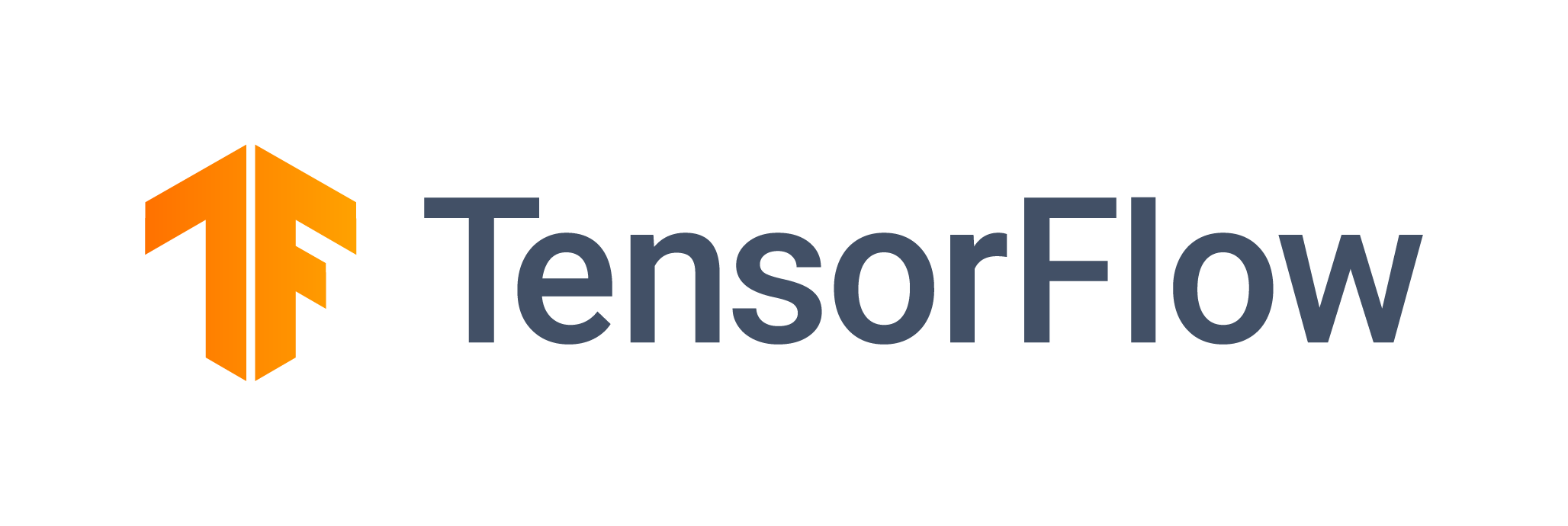# Train a TensorFlow Model (GPU)

Use TensorFlow to train a neural network using a GPU## Overview

TensorFlow is a popular, powerful framework for deep learning used by data scientists across industries.

In this example, you’ll train Resnet50 architecture to identify different species of birds. This dataset consists of 40,000+ images of birds and has been taken from kaggle.

``````import tensorflow as tf
import keras
import time
import matplotlib.pyplot as plt
``````

The dataset originally had 285 classes. We have taken a subset of this data, which has 61 classes. The data is stored in AWS S3. The first time you run this job, you’ll need to download the training and test data in the code chunk below:

``````import s3fs

s3 = s3fs.S3FileSystem(anon=True)
_ = s3.get(
rpath="s3://saturn-public-data/100-bird-species/100-bird-species/*/*/*.jpg",
lpath="dataset/birds/",
)
``````

Our dataset has already neatly separated training, test, and validation samples. In the code below, we construct a Keras data object for the training and validation sets using `keras.preprocessing.image_dataset_from_directory`. We chose the Adam optimizer and set the learning rate to 0.02. We train our classifier using the ResNet50 architecture, which has 48 Convolution layers along with 1 MaxPool and 1 Average Pool layer. It learns from each image, updates the model gradients, and gradually improves its performance. The model is compiled, trained, and saved in `model/keras_single/`.

``````def train_model_fit(n_epochs, base_lr, batchsize, classes):

model = tf.keras.applications.ResNet50(include_top=True, weights=None, classes=classes)

# Data
train_ds = (
tf.keras.preprocessing.image_dataset_from_directory(
"dataset/birds/train", image_size=(224, 224), batch_size=batchsize
)
.prefetch(2)
.cache()
.shuffle(1000)
)
# printing sample birds images
for birds, labels in train_ds.take(1):
plt.figure(figsize=(18, 18))
for i in range(9):
plt.subplot(3, 3, i + 1)
plt.imshow(birds[i].numpy().astype("uint8"))
plt.axis("off")
plt.show()

valid_ds = tf.keras.preprocessing.image_dataset_from_directory(
"dataset/birds/valid", image_size=(224, 224), batch_size=batchsize
).prefetch(2)

model.compile(loss="sparse_categorical_crossentropy", optimizer=optimizer, metrics=["accuracy"])
start = time.time()

model.fit(
train_ds,
epochs=n_epochs,
validation_data=valid_ds,
)
end = time.time() - start
print("model training time", end)

tf.keras.models.save_model(model, "model/keras_single/")
``````

In the code below, we set up the necessary parameters. We run only a few epochs to save time. But once you’ve got this model working, you’ll have all the information you need to build and run bigger TensorFlow models on Saturn Cloud. A single GPU reviews all our batches every epoch. In this case, it sees 64 images per batch x 640 batches x 3 epochs. These batches are all computed serially; the calculations cannot be parallelized because only one processor is utilized.

``````model_params = {"n_epochs": 3, "base_lr": 0.02, "classes": 61, "batchsize": 64}
``````

The code below runs the model training process and saves your trained model object to the Jupyter instance memory. A folder called `model` will be created and populated for you. Also, when you run the training function, you can see some beautiful birds of various species!

``````tester = train_model_fit(**model_params)
``````
``````import tensorflow as tf
import keras
import time
import matplotlib.pyplot as plt

import s3fs

s3 = s3fs.S3FileSystem(anon=True)
_ = s3.get(
rpath="s3://saturn-public-data/100-bird-species/100-bird-species/*/*/*.jpg",
lpath="dataset/birds/",
)

def train_model_fit(n_epochs, base_lr, batchsize, classes):

model = tf.keras.applications.ResNet50(include_top=True, weights=None, classes=classes)

# Data
train_ds = (
tf.keras.preprocessing.image_dataset_from_directory(
"dataset/birds/train", image_size=(224, 224), batch_size=batchsize
)
.prefetch(2)
.cache()
.shuffle(1000)
)
# printing sample birds images
for birds, labels in train_ds.take(1):
plt.figure(figsize=(18, 18))
for i in range(9):
plt.subplot(3, 3, i + 1)
plt.imshow(birds[i].numpy().astype("uint8"))
plt.axis("off")
plt.show()

valid_ds = tf.keras.preprocessing.image_dataset_from_directory(
"dataset/birds/valid", image_size=(224, 224), batch_size=batchsize
).prefetch(2)

model.compile(loss="sparse_categorical_crossentropy", optimizer=optimizer, metrics=["accuracy"])
start = time.time()

model.fit(
train_ds,
epochs=n_epochs,
validation_data=valid_ds,
)
end = time.time() - start
print("model training time", end)

tf.keras.models.save_model(model, "model/keras_single/")

model_params = {"n_epochs": 3, "base_lr": 0.02, "classes": 61, "batchsize": 64}

tester = train_model_fit(**model_params)

``````